# Frank Solutions for Class 9 Maths Chapter 7 Linear Equations

Frank Solutions for Class 9 Maths Chapter 7 Linear Equations contain step-by-step answers in order to help students understand the concepts covered in this chapter thoroughly. The solutions are well-structured by the subject-matter experts at BYJU’S with the aim of helping them in exam preparation. Students are suggested that they refer to these solutions while solving the textbook questions to clear their doubts instantly.

Chapter 7 of Class 9 Maths discusses all the fundamental concepts of Linear Equations, which are significant from an exam perspective. Students are recommended to practise Frank Solutions to perform well in academics as well as score high in the annual exam. The solutions are available both online and offline modes and can be used whenever required. To achieve good marks in the final exam, students can download Frank Solutions for Class 9 Maths Chapter 7 Linear Equations PDF from the link provided below.

## Frank Solutions for Class 9 Maths Chapter 7 Linear Equations Download PDF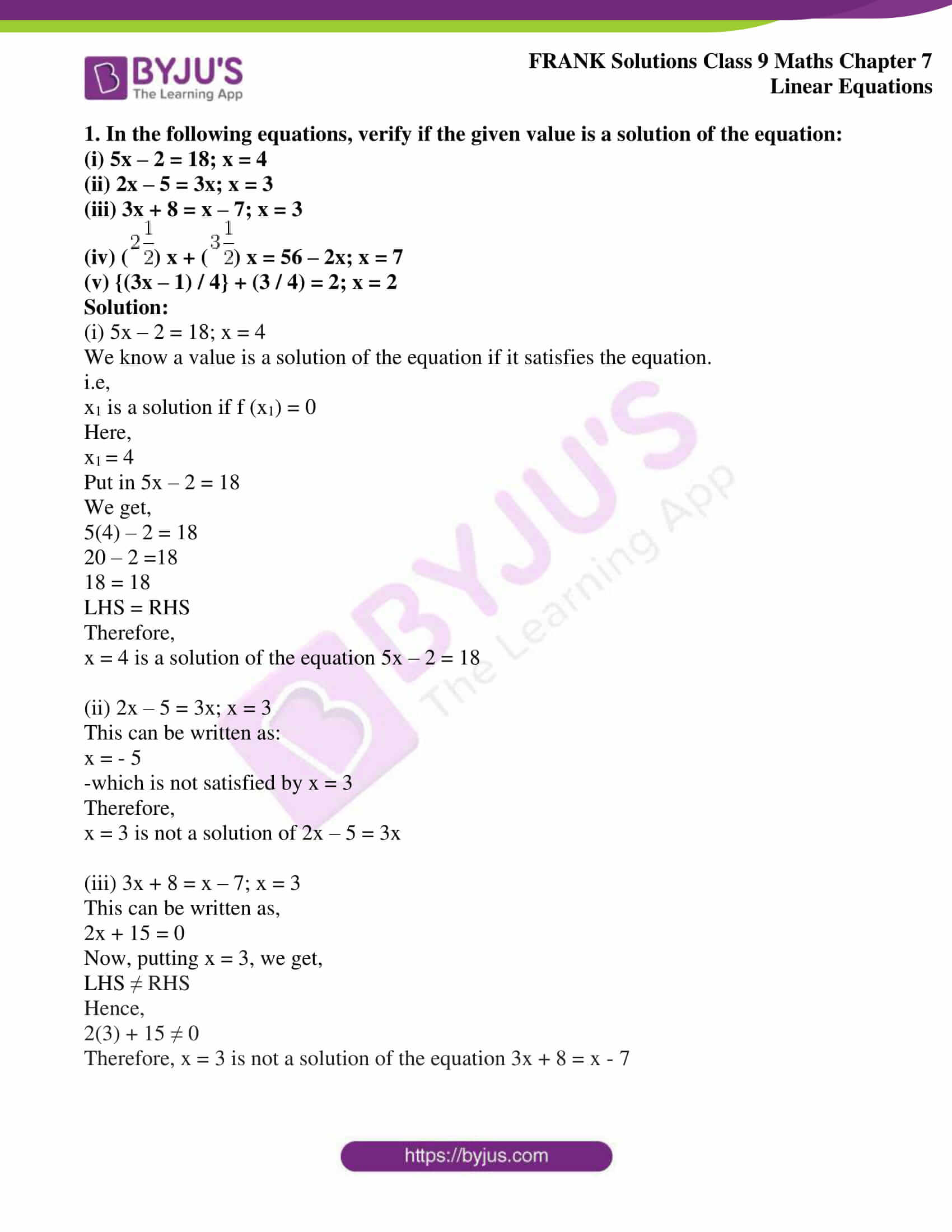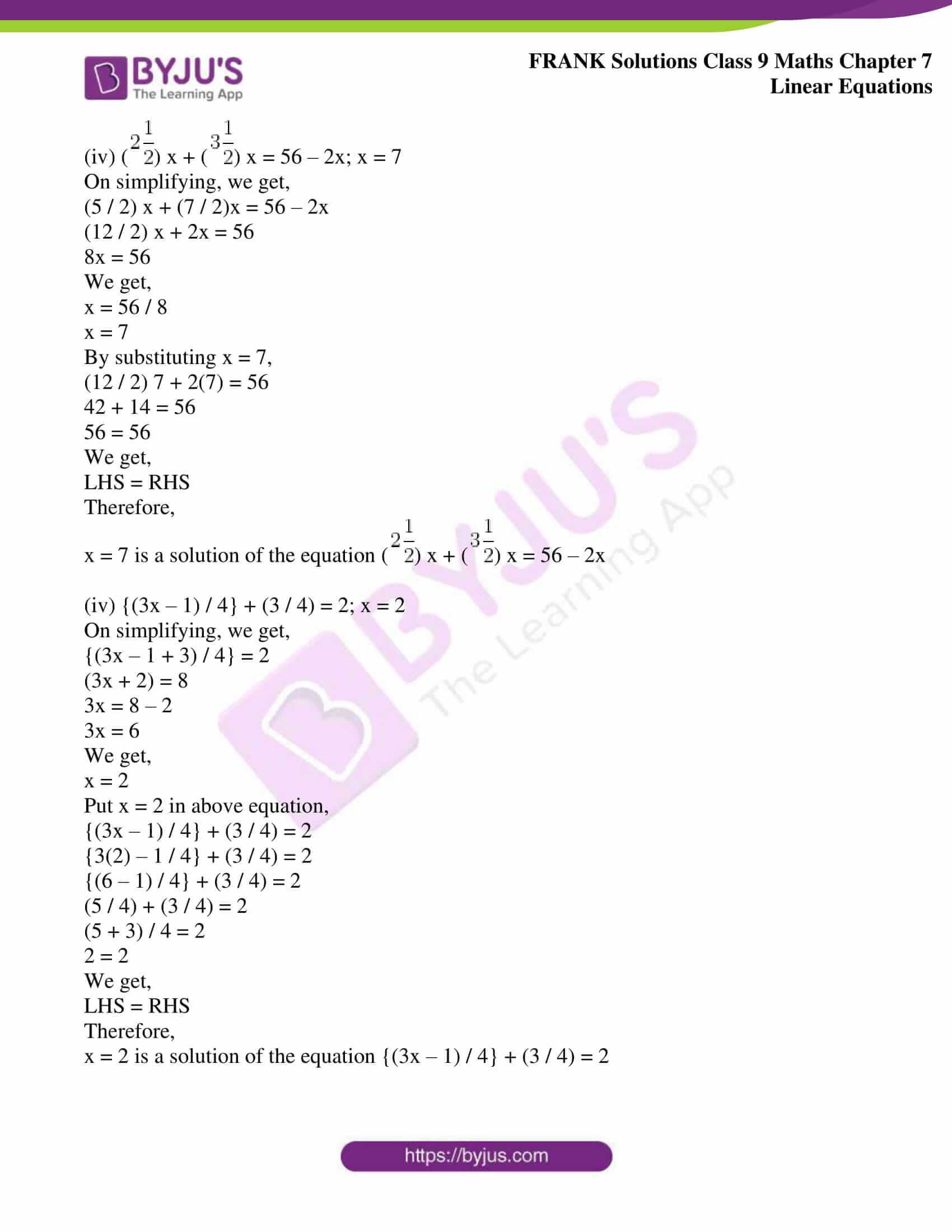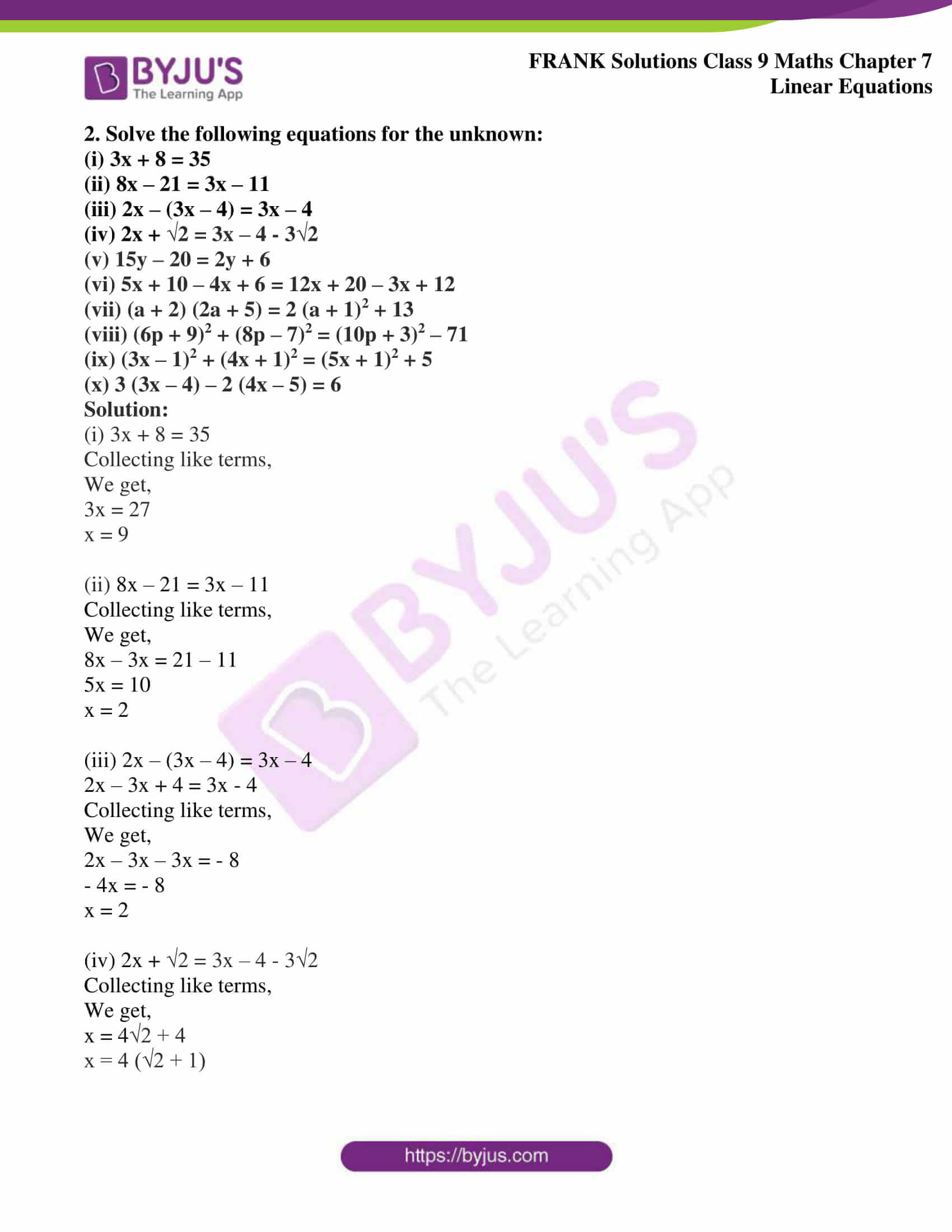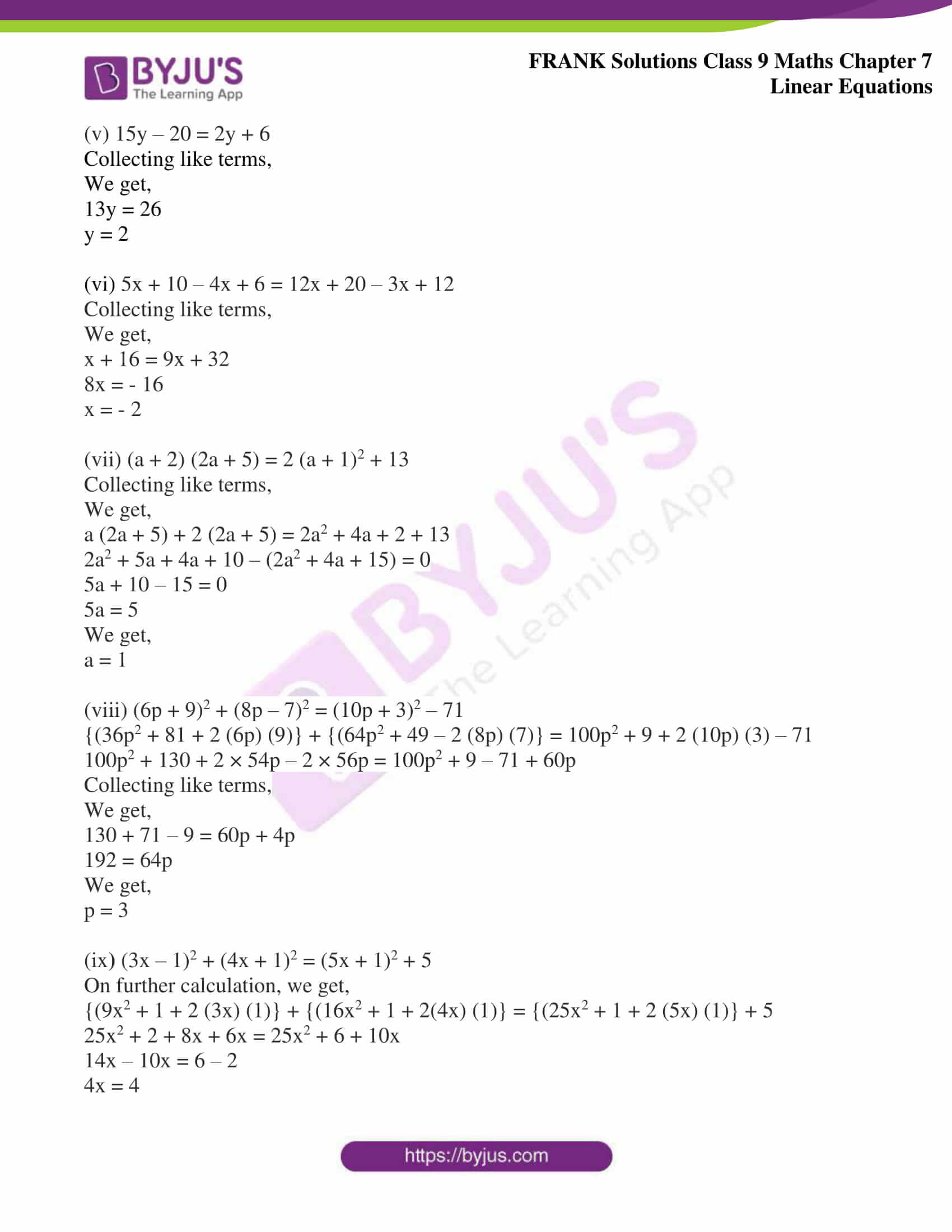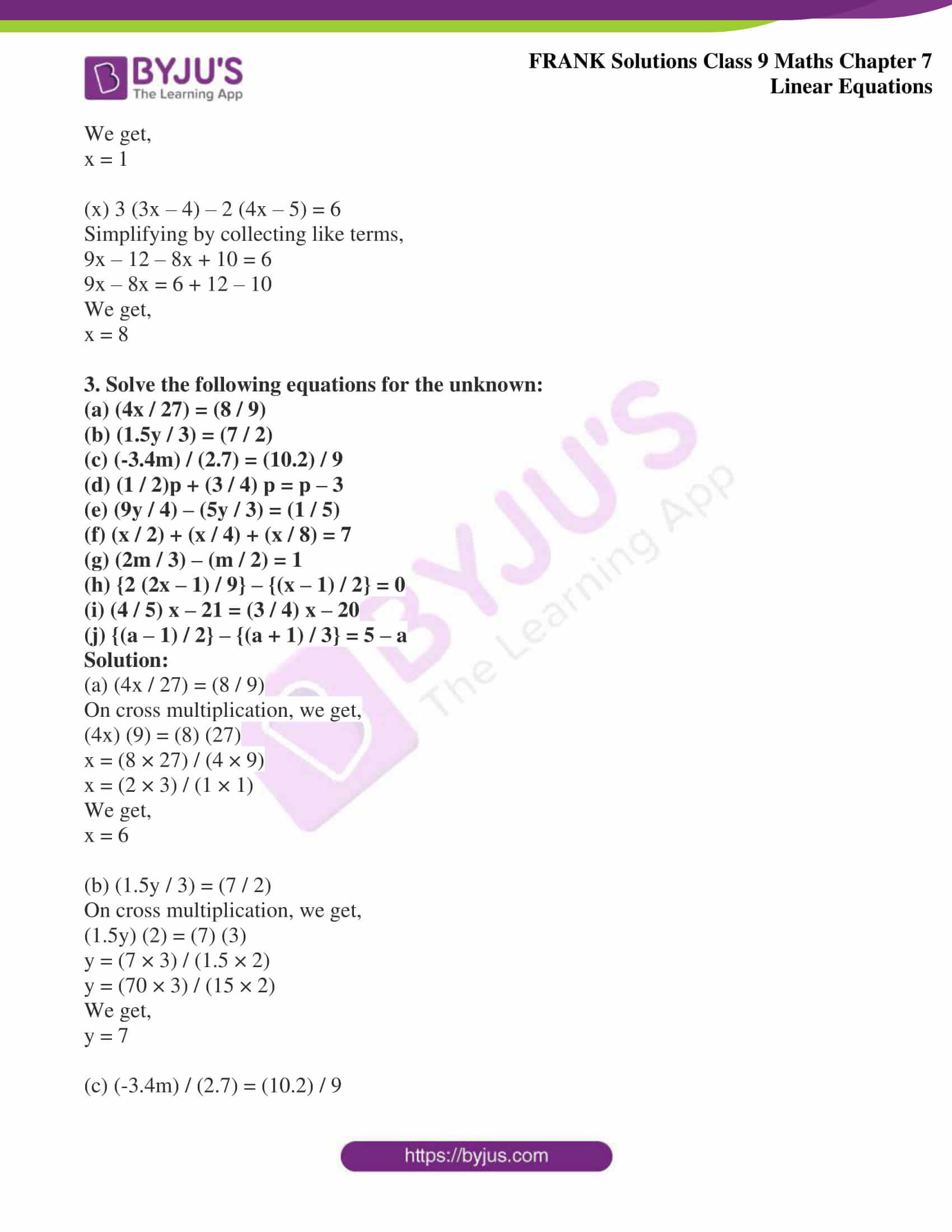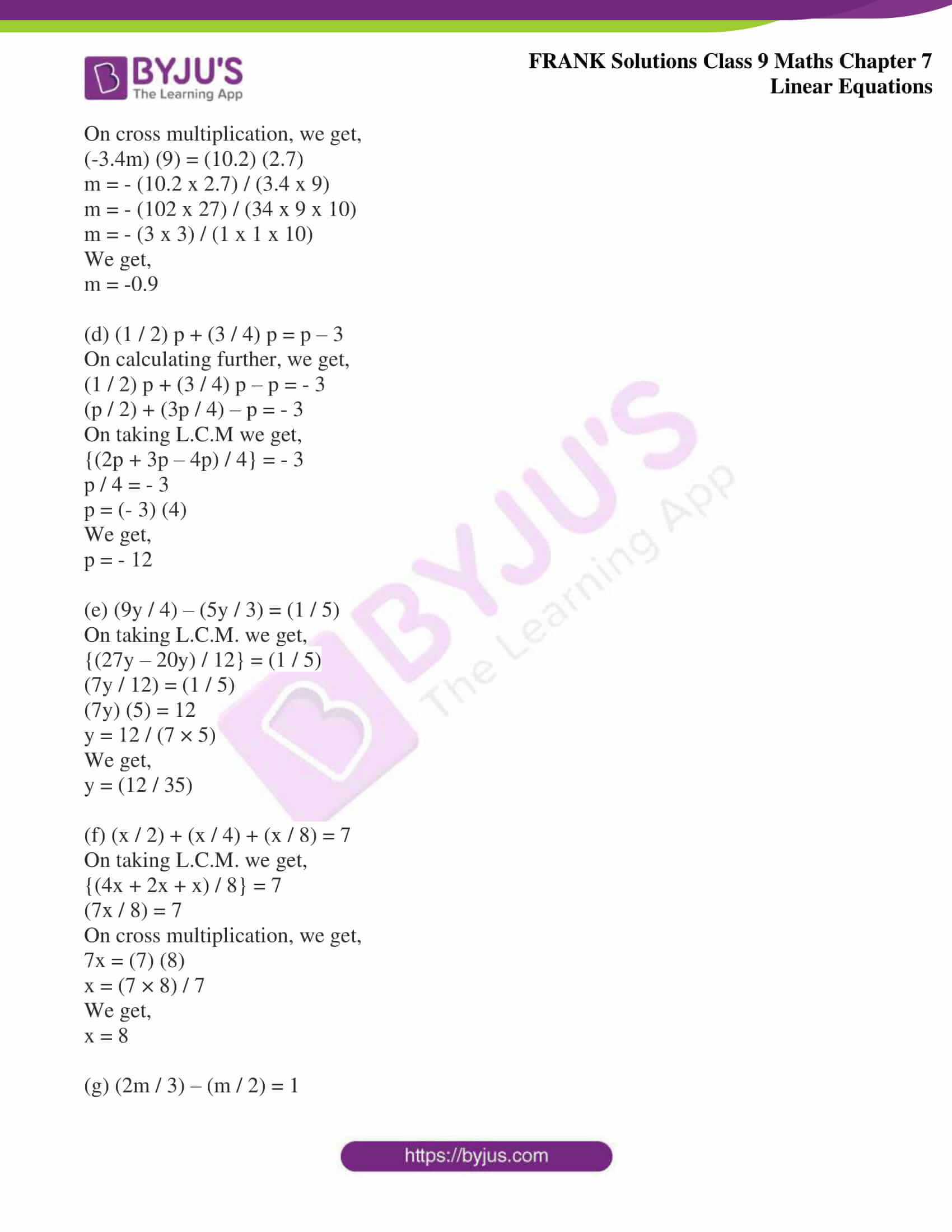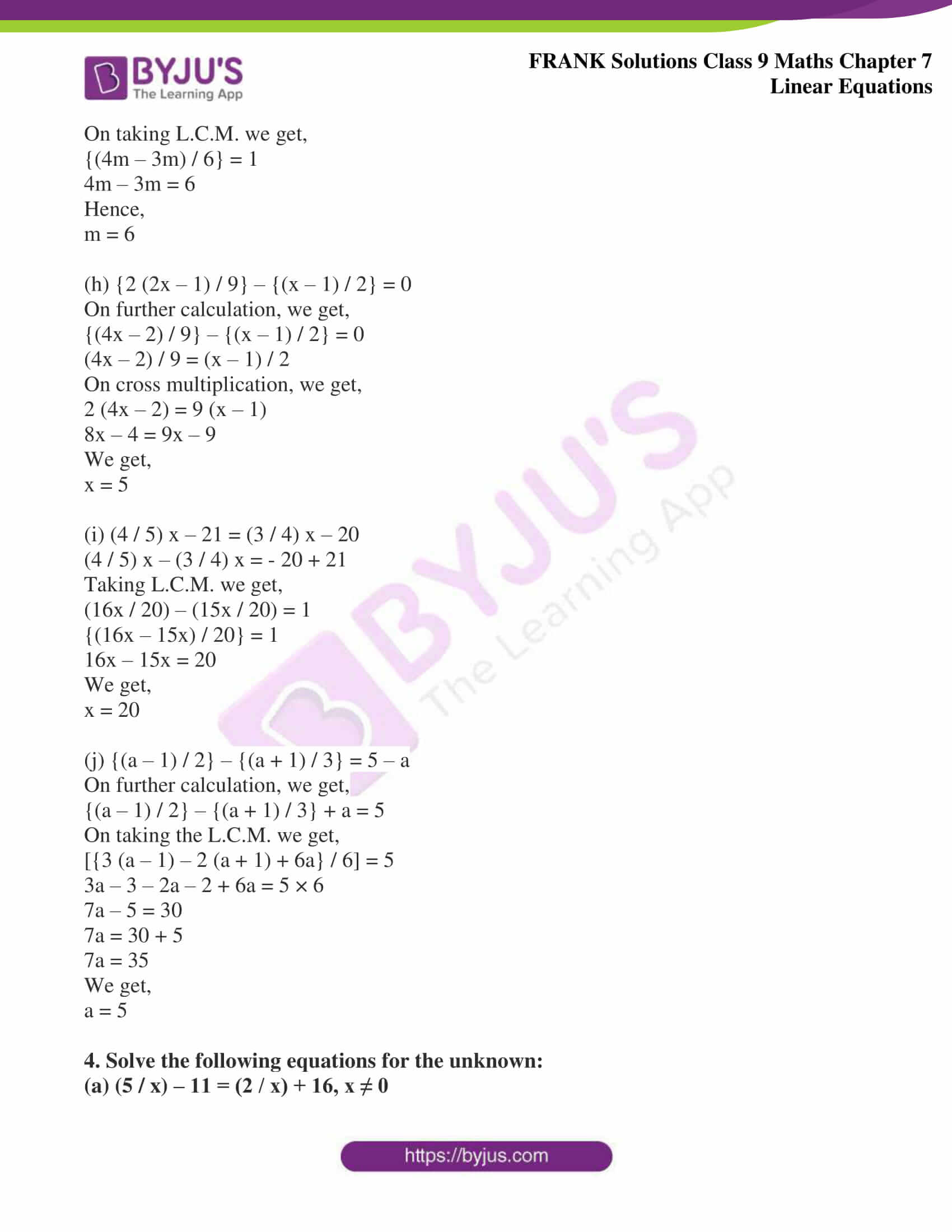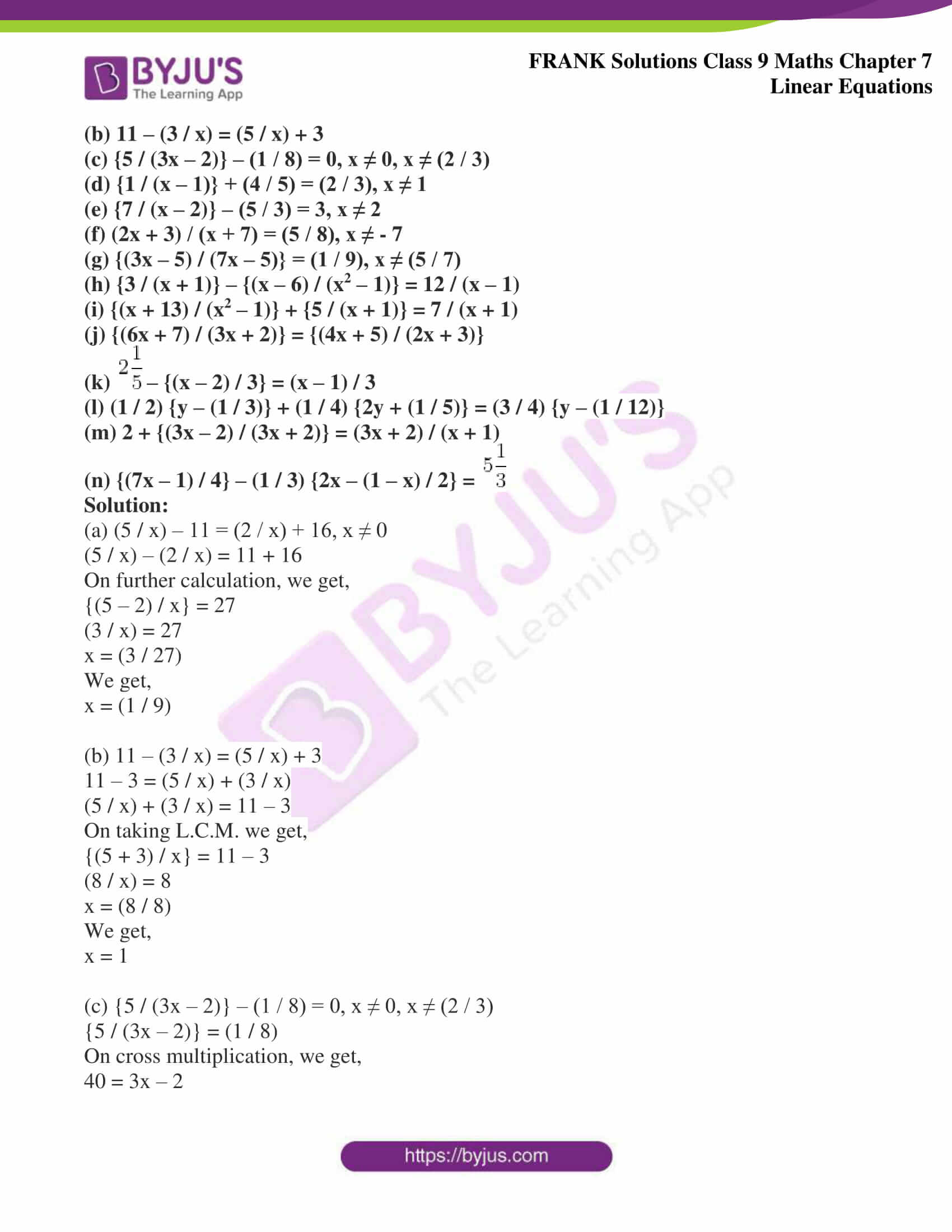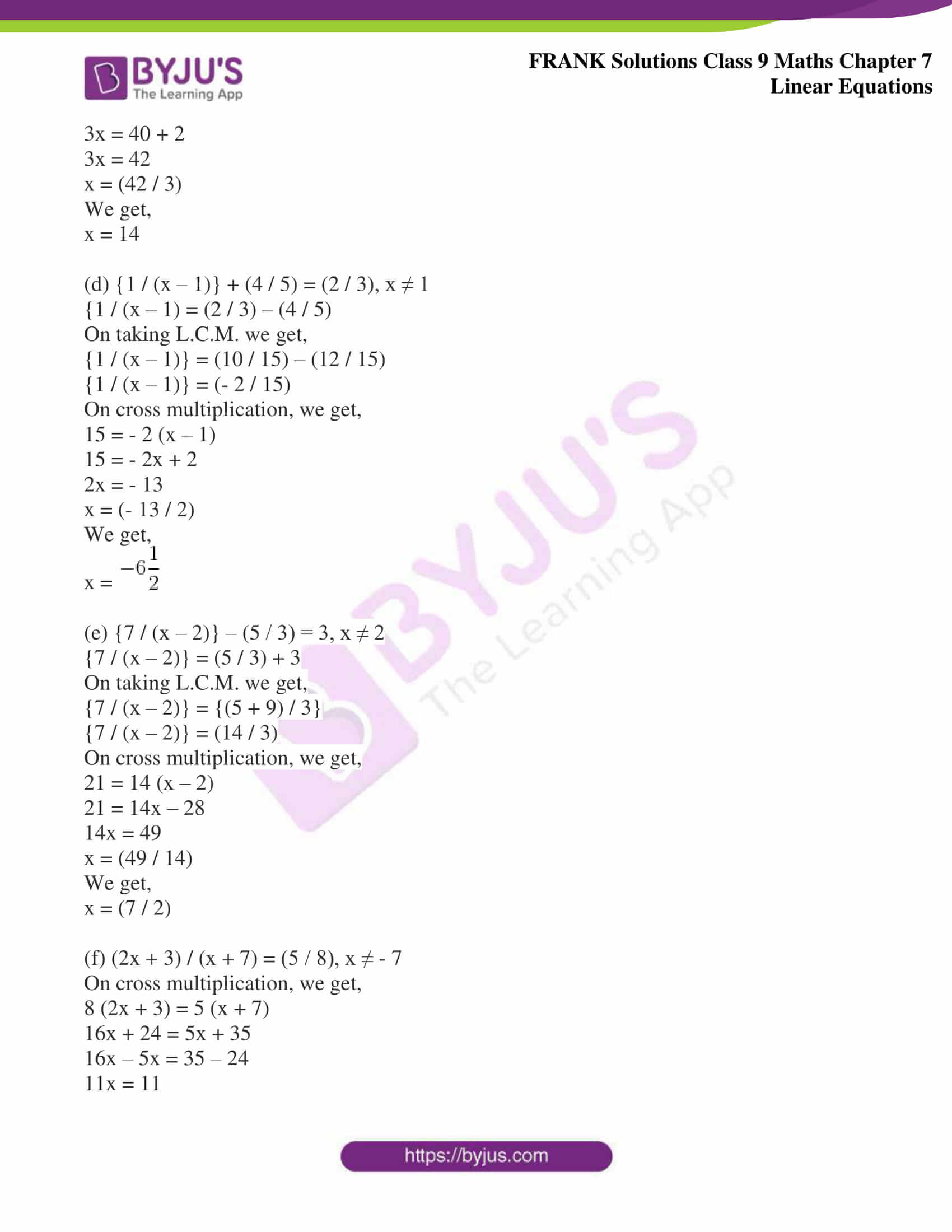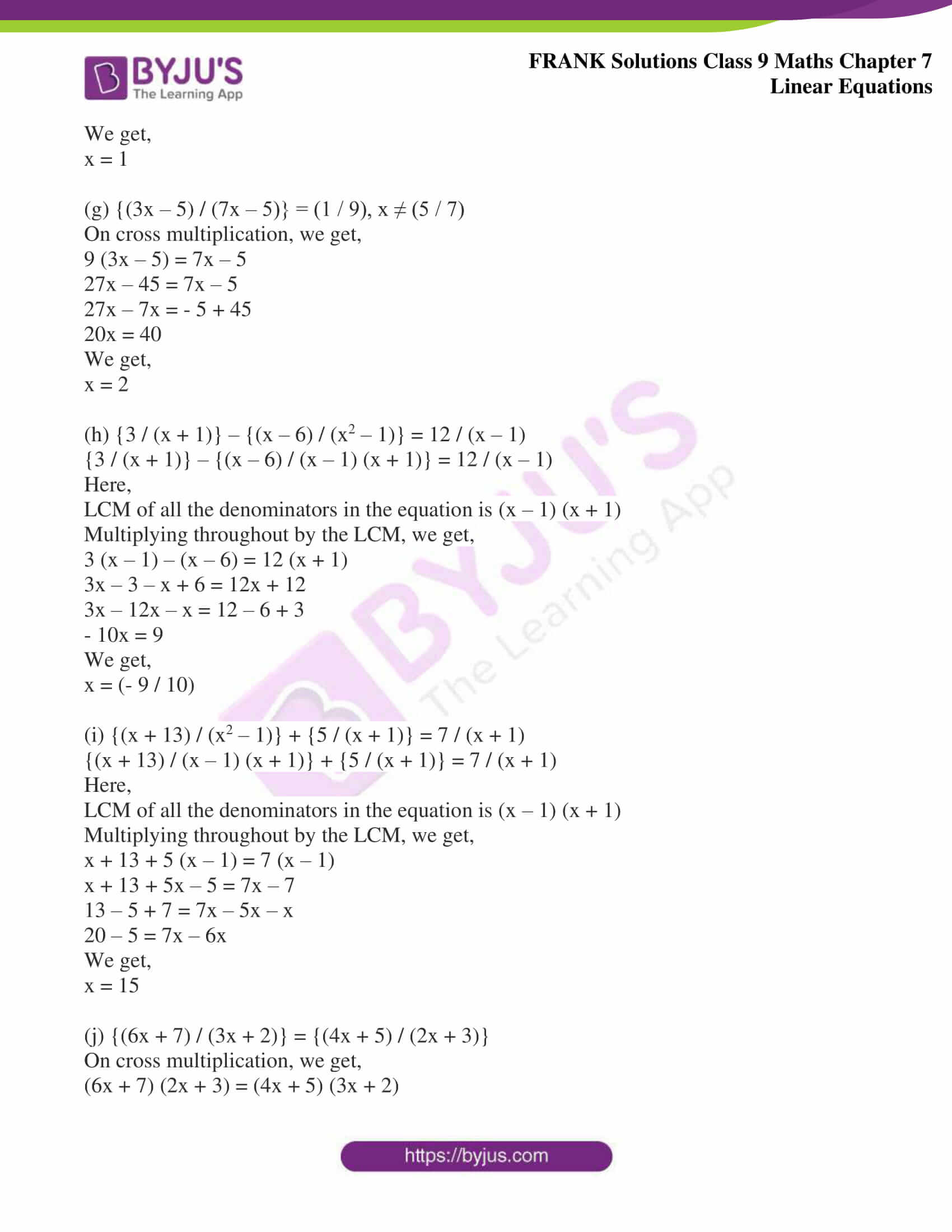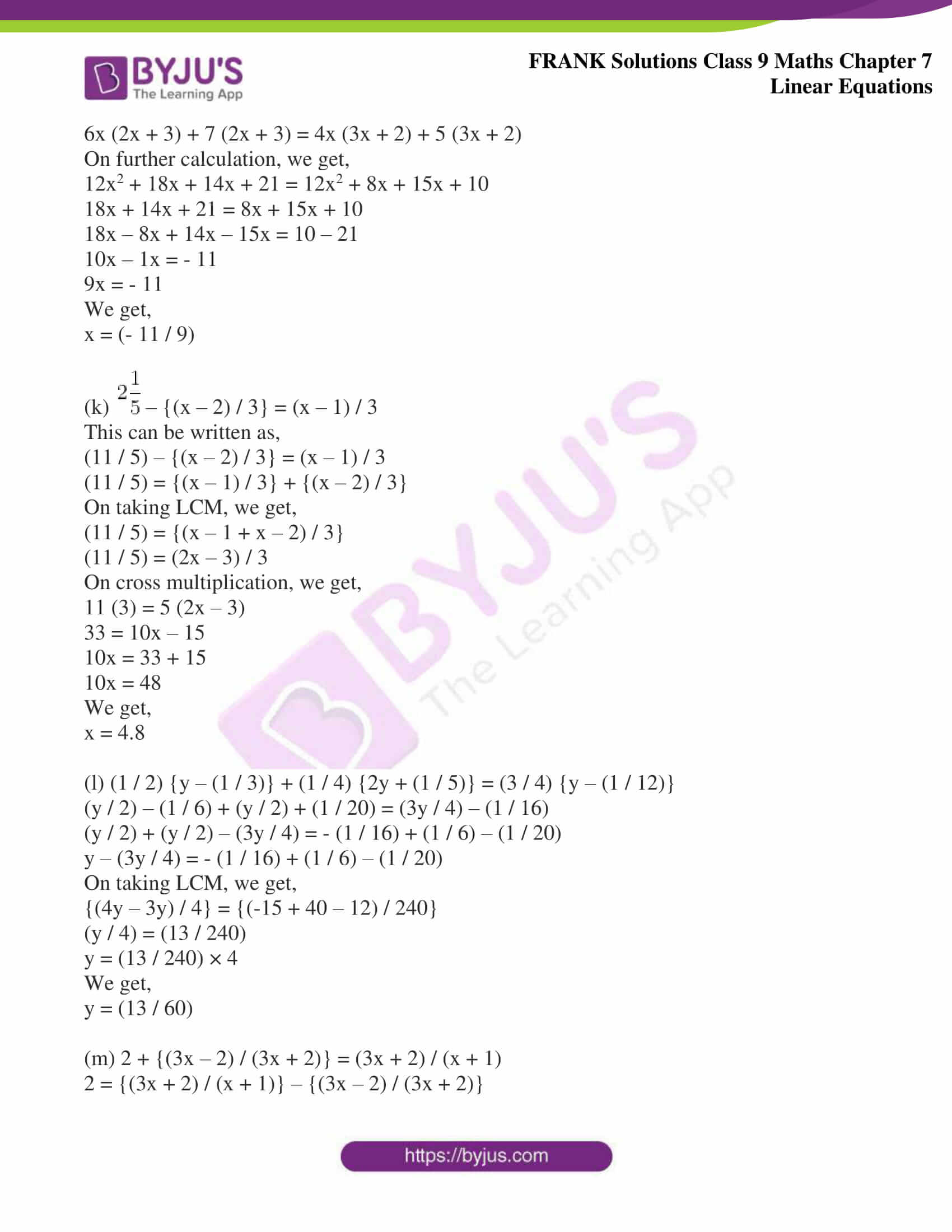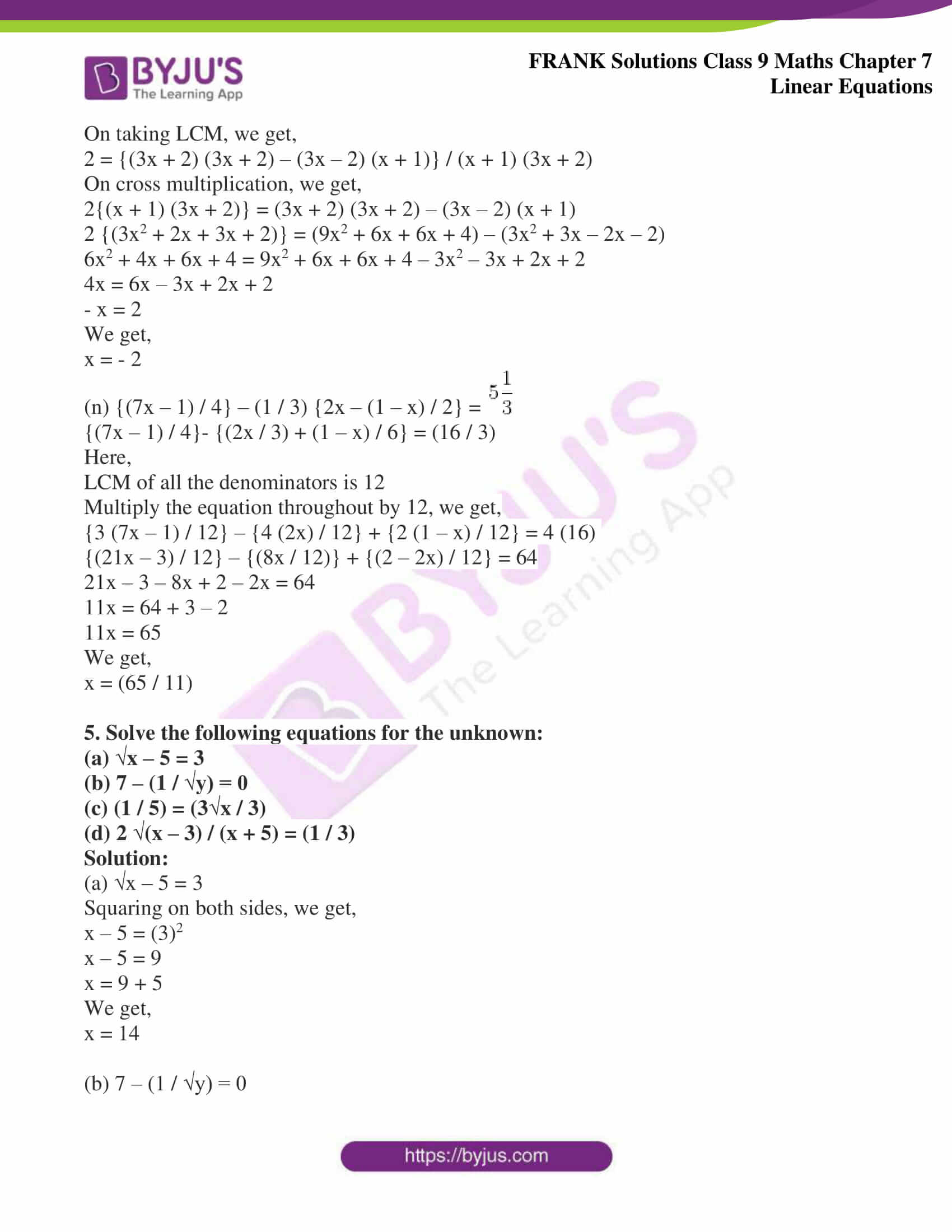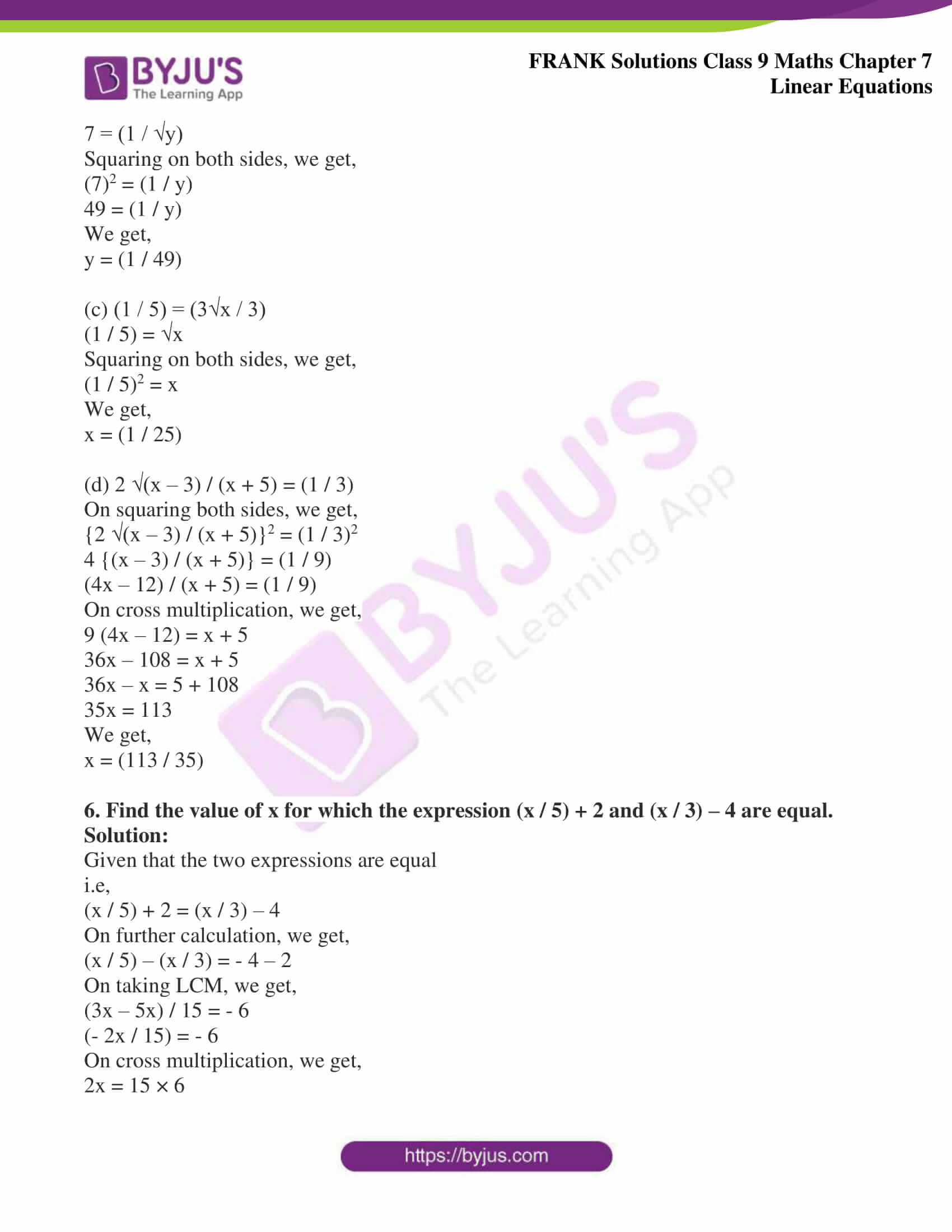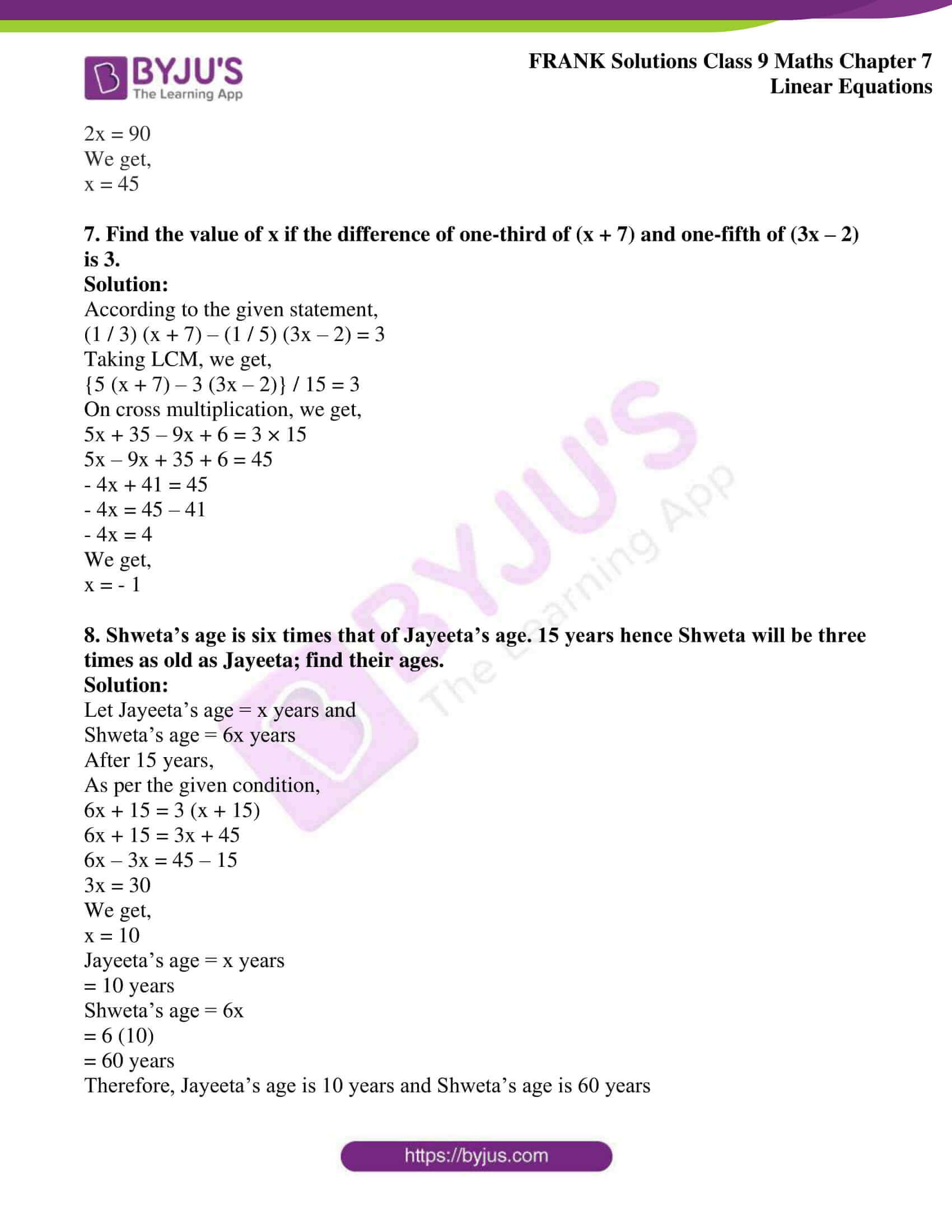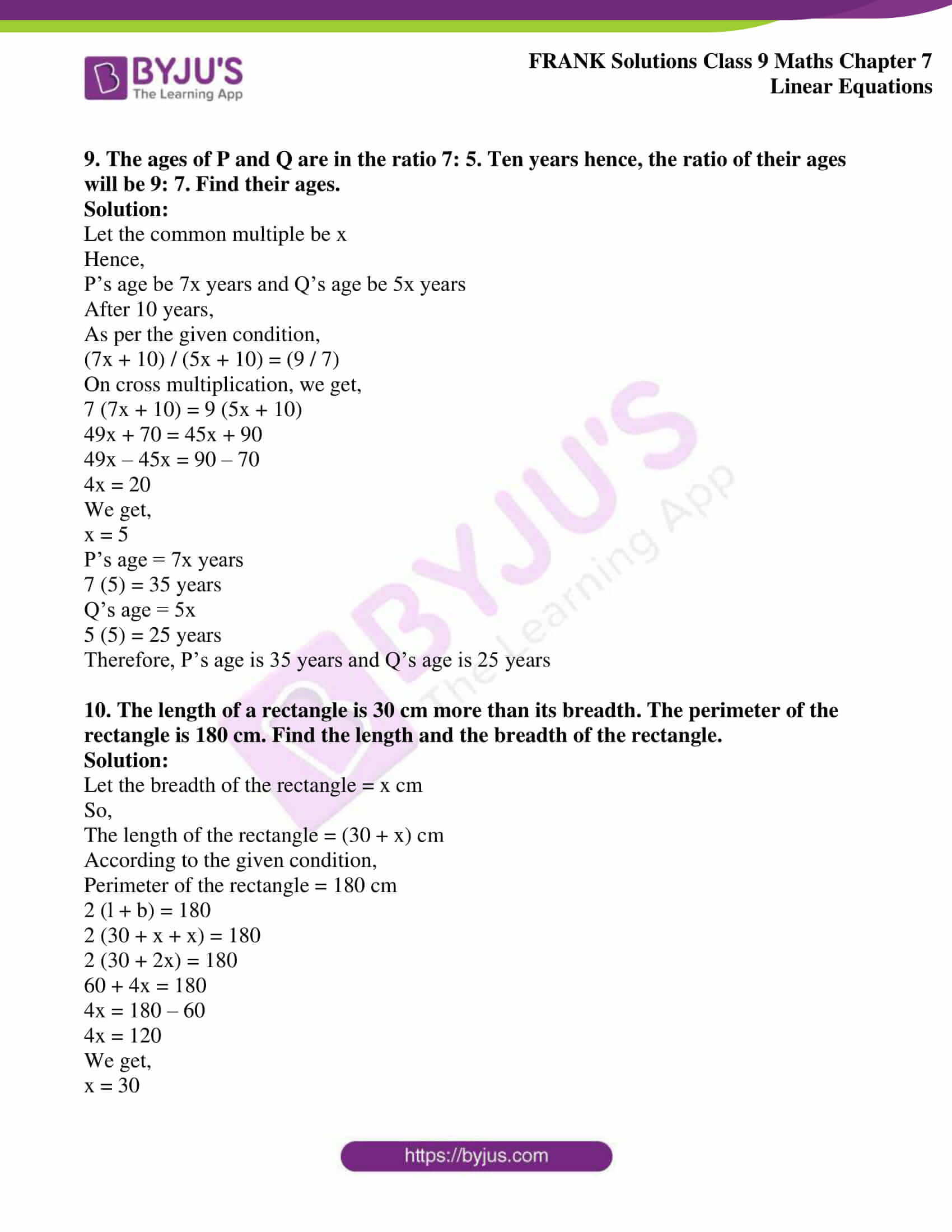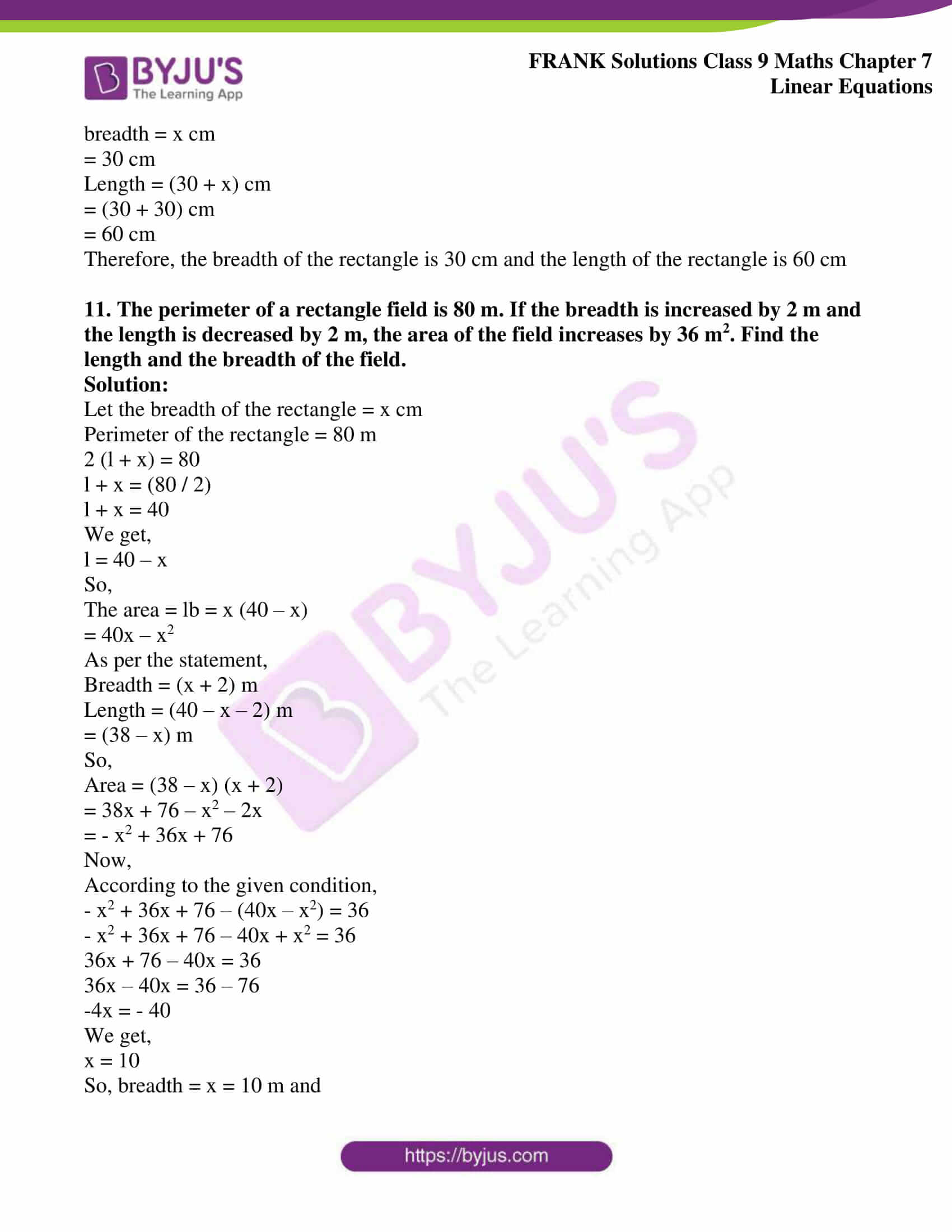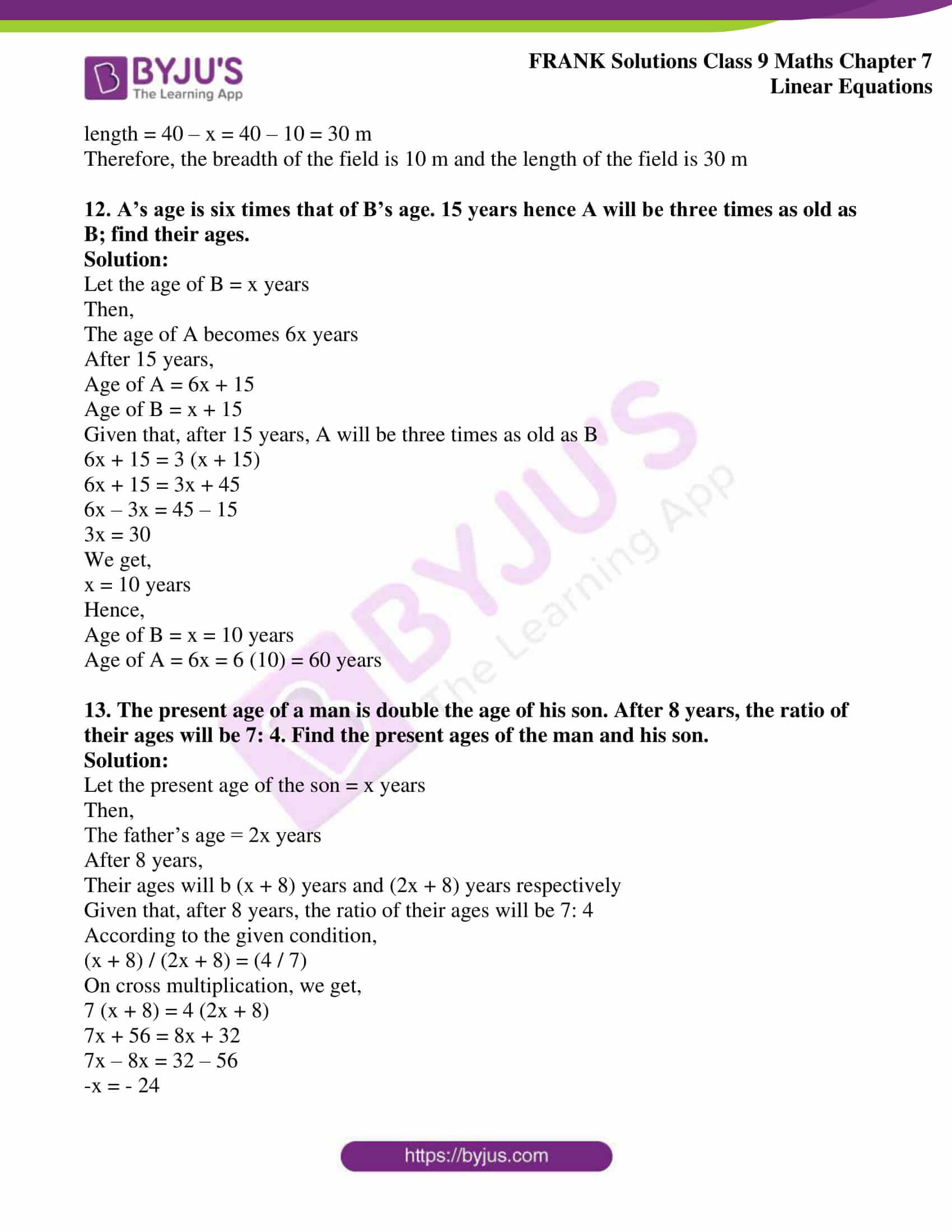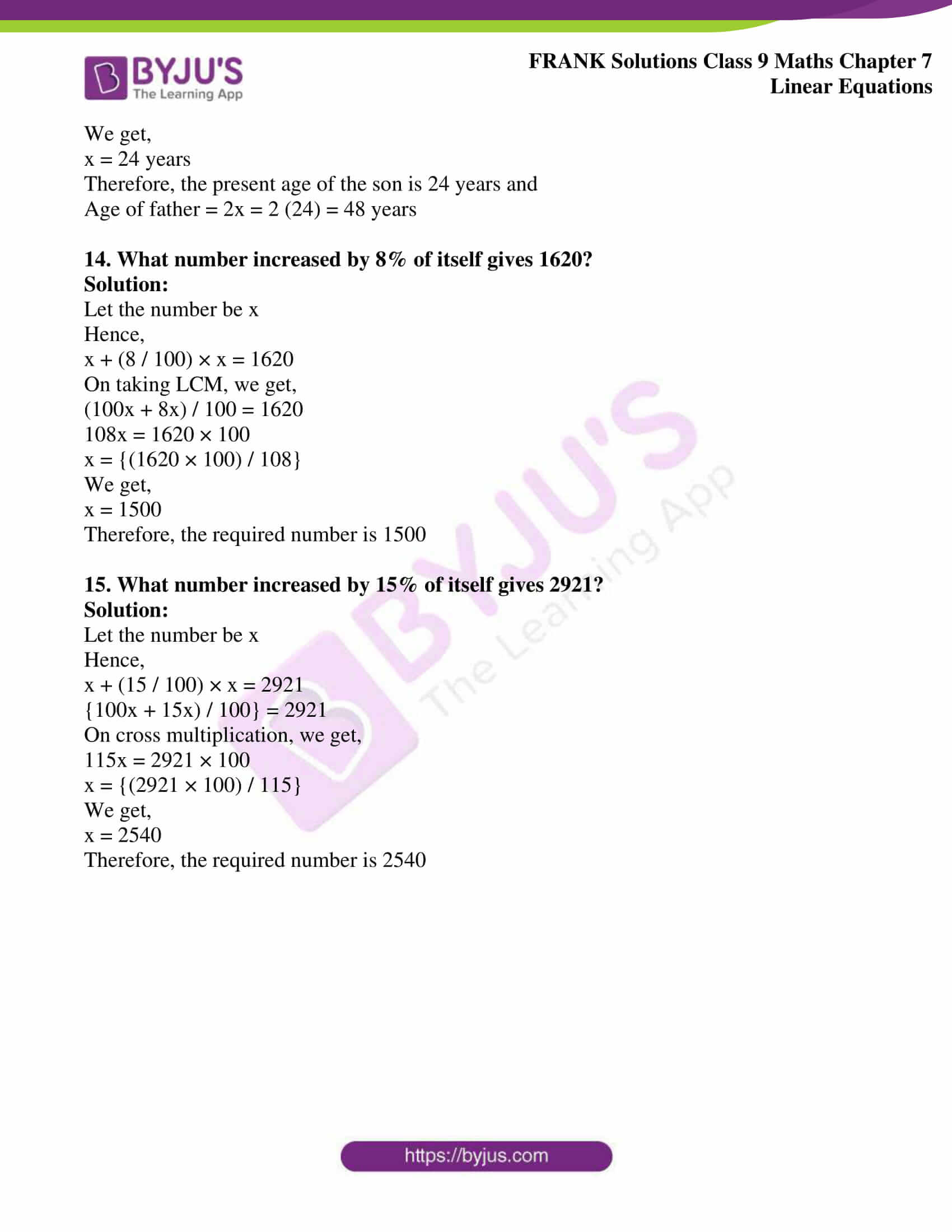## Access Frank Solutions for Class 9 Maths Chapter 7 Linear Equations

1. In the following equations, verify if the given value is a solution of the equation:

(i) 5x – 2 = 18; x = 4

(ii) 2x – 5 = 3x; x = 3

(iii) 3x + 8 = x – 7; x = 3

(iv) (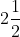) x + (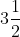) x = 56 – 2x; x = 7

(v) {(3x – 1) / 4} + (3 / 4) = 2; x = 2

Solution:

(i) 5x – 2 = 18; x = 4

We know that a value is a solution of the equation if it satisfies the equation.

i.e,

x1 is a solution if f (x1) = 0

Here,

x1 = 4

Put in 5x – 2 = 18

We get,

5(4) – 2 = 18

20 – 2 =18

18 = 18

LHS = RHS

Therefore,

x = 4 is a solution of equation 5x – 2 = 18

(ii) 2x – 5 = 3x; x = 3

This can be written as:

x = – 5

-which is not satisfied by x = 3

Therefore,

x = 3 is not a solution of 2x – 5 = 3x

(iii) 3x + 8 = x – 7; x = 3

This can be written as,

2x + 15 = 0

Now, putting x = 3, we get,

LHS ≠ RHS

Hence,

2(3) + 15 ≠ 0

Therefore, x = 3 is not a solution of the equation 3x + 8 = x – 7

(iv) () x + () x = 56 – 2x; x = 7

On simplifying, we get,

(5 / 2) x + (7 / 2)x = 56 – 2x

(12 / 2) x + 2x = 56

8x = 56

We get,

x = 56 / 8

x = 7

By substituting x = 7,

(12 / 2) 7 + 2(7) = 56

42 + 14 = 56

56 = 56

We get,

LHS = RHS

Therefore,

x = 7 is a solution of the equation () x + () x = 56 – 2x

(iv) {(3x – 1) / 4} + (3 / 4) = 2; x = 2

On simplifying, we get,

{(3x – 1 + 3) / 4} = 2

(3x + 2) = 8

3x = 8 – 2

3x = 6

We get,

x = 2

Put x = 2 in the above equation,

{(3x – 1) / 4} + (3 / 4) = 2

{3(2) – 1 / 4} + (3 / 4) = 2

{(6 – 1) / 4} + (3 / 4) = 2

(5 / 4) + (3 / 4) = 2

(5 + 3) / 4 = 2

2 = 2

We get,

LHS = RHS

Therefore,

x = 2 is a solution of the equation {(3x – 1) / 4} + (3 / 4) = 2

2. Solve the following equations for the unknown:

(i) 3x + 8 = 35

(ii) 8x – 21 = 3x – 11

(iii) 2x – (3x – 4) = 3x – 4

(iv) 2x + √2 = 3x – 4 – 3√2

(v) 15y – 20 = 2y + 6

(vi) 5x + 10 – 4x + 6 = 12x + 20 – 3x + 12

(vii) (a + 2) (2a + 5) = 2 (a + 1)2 + 13

(viii) (6p + 9)2 + (8p – 7)2 = (10p + 3)2 – 71

(ix) (3x – 1)2 + (4x + 1)2 = (5x + 1)2 + 5

(x) 3 (3x – 4) – 2 (4x – 5) = 6

Solution:

(i) 3x + 8 = 35

Collecting like terms,

We get,

3x = 27

x = 9

(ii) 8x – 21 = 3x – 11

Collecting like terms,

We get,

8x – 3x = 21 – 11

5x = 10

x = 2

(iii) 2x – (3x – 4) = 3x – 4

2x – 3x + 4 = 3x – 4

Collecting like terms,

We get,

2x – 3x – 3x = – 8

– 4x = – 8

x = 2

(iv) 2x + √2 = 3x – 4 – 3√2

Collecting like terms,

We get,

x = 4√2 + 4

x = 4 (√2 + 1)

(v) 15y – 20 = 2y + 6

Collecting like terms,

We get,

13y = 26

y = 2

(vi) 5x + 10 – 4x + 6 = 12x + 20 – 3x + 12

Collecting like terms,

We get,

x + 16 = 9x + 32

8x = – 16

x = – 2

(vii) (a + 2) (2a + 5) = 2 (a + 1)2 + 13

Collecting like terms,

We get,

a (2a + 5) + 2 (2a + 5) = 2a2 + 4a + 2 + 13

2a2 + 5a + 4a + 10 – (2a2 + 4a + 15) = 0

5a + 10 – 15 = 0

5a = 5

We get,

a = 1

(viii) (6p + 9)2 + (8p – 7)2 = (10p + 3)2 – 71

{(36p2 + 81 + 2 (6p) (9)} + {(64p2 + 49 – 2 (8p) (7)} = 100p2 + 9 + 2 (10p) (3) – 71

100p2 + 130 + 2 × 54p – 2 × 56p = 100p2 + 9 – 71 + 60p

Collecting like terms,

We get,

130 + 71 – 9 = 60p + 4p

192 = 64p

We get,

p = 3

(ix) (3x – 1)2 + (4x + 1)2 = (5x + 1)2 + 5

On further calculation, we get,

{(9x2 + 1 + 2 (3x) (1)} + {(16x2 + 1 + 2(4x) (1)} = {(25x2 + 1 + 2 (5x) (1)} + 5

25x2 + 2 + 8x + 6x = 25x2 + 6 + 10x

14x – 10x = 6 – 2

4x = 4

We get,

x = 1

(x) 3 (3x – 4) – 2 (4x – 5) = 6

Simplifying by collecting like terms,

9x – 12 – 8x + 10 = 6

9x – 8x = 6 + 12 – 10

We get,

x = 8

3. Solve the following equations for the unknown:

(a) (4x / 27) = (8 / 9)

(b) (1.5y / 3) = (7 / 2)

(c) (-3.4m) / (2.7) = (10.2) / 9

(d) (1 / 2)p + (3 / 4) p = p – 3

(e) (9y / 4) – (5y / 3) = (1 / 5)

(f) (x / 2) + (x / 4) + (x / 8) = 7

(g) (2m / 3) – (m / 2) = 1

(h) {2 (2x – 1) / 9} – {(x – 1) / 2} = 0

(i) (4 / 5) x – 21 = (3 / 4) x – 20

(j) {(a – 1) / 2} – {(a + 1) / 3} = 5 – a

Solution:

(a) (4x / 27) = (8 / 9)

On cross multiplication, we get,

(4x) (9) = (8) (27)

x = (8 × 27) / (4 × 9)

x = (2 × 3) / (1 × 1)

We get,

x = 6

(b) (1.5y / 3) = (7 / 2)

On cross multiplication, we get,

(1.5y) (2) = (7) (3)

y = (7 × 3) / (1.5 × 2)

y = (70 × 3) / (15 × 2)

We get,

y = 7

(c) (-3.4m) / (2.7) = (10.2) / 9

On cross multiplication, we get,

(-3.4m) (9) = (10.2) (2.7)

m = – (10.2 x 2.7) / (3.4 x 9)

m = – (102 x 27) / (34 x 9 x 10)

m = – (3 x 3) / (1 x 1 x 10)

We get,

m = -0.9

(d) (1 / 2) p + (3 / 4) p = p – 3

On calculating further, we get,

(1 / 2) p + (3 / 4) p – p = – 3

(p / 2) + (3p / 4) – p = – 3

On taking L.C.M, we get,

{(2p + 3p – 4p) / 4} = – 3

p / 4 = – 3

p = (- 3) (4)

We get,

p = – 12

(e) (9y / 4) – (5y / 3) = (1 / 5)

On taking L.C.M., we get,

{(27y – 20y) / 12} = (1 / 5)

(7y / 12) = (1 / 5)

(7y) (5) = 12

y = 12 / (7 × 5)

We get,

y = (12 / 35)

(f) (x / 2) + (x / 4) + (x / 8) = 7

On taking L.C.M., we get,

{(4x + 2x + x) / 8} = 7

(7x / 8) = 7

On cross multiplication, we get,

7x = (7) (8)

x = (7 × 8) / 7

We get,

x = 8

(g) (2m / 3) – (m / 2) = 1

On taking L.C.M., we get,

{(4m – 3m) / 6} = 1

4m – 3m = 6

Hence,

m = 6

(h) {2 (2x – 1) / 9} – {(x – 1) / 2} = 0

On further calculation, we get,

{(4x – 2) / 9} – {(x – 1) / 2} = 0

(4x – 2) / 9 = (x – 1) / 2

On cross multiplication, we get,

2 (4x – 2) = 9 (x – 1)

8x – 4 = 9x – 9

We get,

x = 5

(i) (4 / 5) x – 21 = (3 / 4) x – 20

(4 / 5) x – (3 / 4) x = – 20 + 21

Taking L.C.M., we get,

(16x / 20) – (15x / 20) = 1

{(16x – 15x) / 20} = 1

16x – 15x = 20

We get,

x = 20

(j) {(a – 1) / 2} – {(a + 1) / 3} = 5 – a

On further calculation, we get,

{(a – 1) / 2} – {(a + 1) / 3} + a = 5

On taking the L.C.M., we get,

[{3 (a – 1) – 2 (a + 1) + 6a} / 6] = 5

3a – 3 – 2a – 2 + 6a = 5 × 6

7a – 5 = 30

7a = 30 + 5

7a = 35

We get,

a = 5

4. Solve the following equations for the unknown:

(a) (5 / x) – 11 = (2 / x) + 16, x ≠ 0

(b) 11 – (3 / x) = (5 / x) + 3

(c) {5 / (3x – 2)} – (1 / 8) = 0, x ≠ 0, x ≠ (2 / 3)

(d) {1 / (x – 1)} + (4 / 5) = (2 / 3), x ≠ 1

(e) {7 / (x – 2)} – (5 / 3) = 3, x ≠ 2

(f) (2x + 3) / (x + 7) = (5 / 8), x ≠ – 7

(g) {(3x – 5) / (7x – 5)} = (1 / 9), x ≠ (5 / 7)

(h) {3 / (x + 1)} – {(x – 6) / (x2 – 1)} = 12 / (x – 1)

(i) {(x + 13) / (x2 – 1)} + {5 / (x + 1)} = 7 / (x + 1)

(j) {(6x + 7) / (3x + 2)} = {(4x + 5) / (2x + 3)}

(k)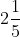– {(x – 2) / 3} = (x – 1) / 3

(l) (1 / 2) {y – (1 / 3)} + (1 / 4) {2y + (1 / 5)} = (3 / 4) {y – (1 / 12)}

(m) 2 + {(3x – 2) / (3x + 2)} = (3x + 2) / (x + 1)

(n) {(7x – 1) / 4} – (1 / 3) {2x – (1 – x) / 2} =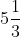Solution:

(a) (5 / x) – 11 = (2 / x) + 16, x ≠ 0

(5 / x) – (2 / x) = 11 + 16

On further calculation, we get,

{(5 – 2) / x} = 27

(3 / x) = 27

x = (3 / 27)

We get,

x = (1 / 9)

(b) 11 – (3 / x) = (5 / x) + 3

11 – 3 = (5 / x) + (3 / x)

(5 / x) + (3 / x) = 11 – 3

On taking L.C.M., we get,

{(5 + 3) / x} = 11 – 3

(8 / x) = 8

x = (8 / 8)

We get,

x = 1

(c) {5 / (3x – 2)} – (1 / 8) = 0, x ≠ 0, x ≠ (2 / 3)

{5 / (3x – 2)} = (1 / 8)

On cross multiplication, we get,

40 = 3x – 2

3x = 40 + 2

3x = 42

x = (42 / 3)

We get,

x = 14

(d) {1 / (x – 1)} + (4 / 5) = (2 / 3), x ≠ 1

{1 / (x – 1) = (2 / 3) – (4 / 5)

On taking L.C.M., we get,

{1 / (x – 1)} = (10 / 15) – (12 / 15)

{1 / (x – 1)} = (- 2 / 15)

On cross multiplication, we get,

15 = – 2 (x – 1)

15 = – 2x + 2

2x = – 13

x = (- 13 / 2)

We get,

x =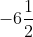(e) {7 / (x – 2)} – (5 / 3) = 3, x ≠ 2

{7 / (x – 2)} = (5 / 3) + 3

On taking L.C.M., we get,

{7 / (x – 2)} = {(5 + 9) / 3}

{7 / (x – 2)} = (14 / 3)

On cross multiplication, we get,

21 = 14 (x – 2)

21 = 14x – 28

14x = 49

x = (49 / 14)

We get,

x = (7 / 2)

(f) (2x + 3) / (x + 7) = (5 / 8), x ≠ – 7

On cross multiplication, we get,

8 (2x + 3) = 5 (x + 7)

16x + 24 = 5x + 35

16x – 5x = 35 – 24

11x = 11

We get,

x = 1

(g) {(3x – 5) / (7x – 5)} = (1 / 9), x ≠ (5 / 7)

On cross multiplication, we get,

9 (3x – 5) = 7x – 5

27x – 45 = 7x – 5

27x – 7x = – 5 + 45

20x = 40

We get,

x = 2

(h) {3 / (x + 1)} – {(x – 6) / (x2 – 1)} = 12 / (x – 1)

{3 / (x + 1)} – {(x – 6) / (x – 1) (x + 1)} = 12 / (x – 1)

Here,

LCM of all the denominators in the equation is (x – 1) (x + 1)

Multiplying throughout by the LCM, we get,

3 (x – 1) – (x – 6) = 12 (x + 1)

3x – 3 – x + 6 = 12x + 12

3x – 12x – x = 12 – 6 + 3

– 10x = 9

We get,

x = (- 9 / 10)

(i) {(x + 13) / (x2 – 1)} + {5 / (x + 1)} = 7 / (x + 1)

{(x + 13) / (x – 1) (x + 1)} + {5 / (x + 1)} = 7 / (x + 1)

Here,

LCM of all the denominators in the equation is (x – 1) (x + 1)

Multiplying throughout by the LCM, we get,

x + 13 + 5 (x – 1) = 7 (x – 1)

x + 13 + 5x – 5 = 7x – 7

13 – 5 + 7 = 7x – 5x – x

20 – 5 = 7x – 6x

We get,

x = 15

(j) {(6x + 7) / (3x + 2)} = {(4x + 5) / (2x + 3)}

On cross multiplication, we get,

(6x + 7) (2x + 3) = (4x + 5) (3x + 2)

6x (2x + 3) + 7 (2x + 3) = 4x (3x + 2) + 5 (3x + 2)

On further calculation, we get,

12x2 + 18x + 14x + 21 = 12x2 + 8x + 15x + 10

18x + 14x + 21 = 8x + 15x + 10

18x – 8x + 14x – 15x = 10 – 21

10x – 1x = – 11

9x = – 11

We get,

x = (- 11 / 9)

(k)– {(x – 2) / 3} = (x – 1) / 3

This can be written as,

(11 / 5) – {(x – 2) / 3} = (x – 1) / 3

(11 / 5) = {(x – 1) / 3} + {(x – 2) / 3}

On taking LCM, we get,

(11 / 5) = {(x – 1 + x – 2) / 3}

(11 / 5) = (2x – 3) / 3

On cross multiplication, we get,

11 (3) = 5 (2x – 3)

33 = 10x – 15

10x = 33 + 15

10x = 48

We get,

x = 4.8

(l) (1 / 2) {y – (1 / 3)} + (1 / 4) {2y + (1 / 5)} = (3 / 4) {y – (1 / 12)}

(y / 2) – (1 / 6) + (y / 2) + (1 / 20) = (3y / 4) – (1 / 16)

(y / 2) + (y / 2) – (3y / 4) = – (1 / 16) + (1 / 6) – (1 / 20)

y – (3y / 4) = – (1 / 16) + (1 / 6) – (1 / 20)

On taking LCM, we get,

{(4y – 3y) / 4} = {(-15 + 40 – 12) / 240}

(y / 4) = (13 / 240)

y = (13 / 240) × 4

We get,

y = (13 / 60)

(m) 2 + {(3x – 2) / (3x + 2)} = (3x + 2) / (x + 1)

2 = {(3x + 2) / (x + 1)} – {(3x – 2) / (3x + 2)}

On taking LCM, we get,

2 = {(3x + 2) (3x + 2) – (3x – 2) (x + 1)} / (x + 1) (3x + 2)

On cross multiplication, we get,

2{(x + 1) (3x + 2)} = (3x + 2) (3x + 2) – (3x – 2) (x + 1)

2 {(3x2 + 2x + 3x + 2)} = (9x2 + 6x + 6x + 4) – (3x2 + 3x – 2x – 2)

6x2 + 4x + 6x + 4 = 9x2 + 6x + 6x + 4 – 3x2 – 3x + 2x + 2

4x = 6x – 3x + 2x + 2

– x = 2

We get,

x = – 2

(n) {(7x – 1) / 4} – (1 / 3) {2x – (1 – x) / 2} ={(7x – 1) / 4}- {(2x / 3) + (1 – x) / 6} = (16 / 3)

Here,

LCM of all the denominators is 12

Multiply the equation throughout by 12, and we get,

{3 (7x – 1) / 12} – {4 (2x) / 12} + {2 (1 – x) / 12} = 4 (16)

{(21x – 3) / 12} – {(8x / 12)} + {(2 – 2x) / 12} = 64

21x – 3 – 8x + 2 – 2x = 64

11x = 64 + 3 – 2

11x = 65

We get,

x = (65 / 11)

5. Solve the following equations for the unknown:

(a) √x – 5 = 3

(b) 7 – (1 / √y) = 0

(c) (1 / 5) = (3√x / 3)

(d) 2 √(x – 3) / (x + 5) = (1 / 3)

Solution:

(a) √x – 5 = 3

Squaring on both sides, we get,

x – 5 = (3)2

x – 5 = 9

x = 9 + 5

We get,

x = 14

(b) 7 – (1 / √y) = 0

7 = (1 / √y)

Squaring on both sides, we get,

(7)2 = (1 / y)

49 = (1 / y)

We get,

y = (1 / 49)

(c) (1 / 5) = (3√x / 3)

(1 / 5) = √x

Squaring on both sides, we get,

(1 / 5)2 = x

We get,

x = (1 / 25)

(d) 2 √(x – 3) / (x + 5) = (1 / 3)

On squaring both sides, we get,

{2 √(x – 3) / (x + 5)}2 = (1 / 3)2

4 {(x – 3) / (x + 5)} = (1 / 9)

(4x – 12) / (x + 5) = (1 / 9)

On cross multiplication, we get,

9 (4x – 12) = x + 5

36x – 108 = x + 5

36x – x = 5 + 108

35x = 113

We get,

x = (113 / 35)

6. Find the value of x for which the expression (x / 5) + 2 and (x / 3) – 4 are equal.

Solution:

Given that the two expressions are equal

i.e,

(x / 5) + 2 = (x / 3) – 4

On further calculation, we get,

(x / 5) – (x / 3) = – 4 – 2

On taking LCM, we get,

(3x – 5x) / 15 = – 6

(- 2x / 15) = – 6

On cross multiplication, we get,

2x = 15 × 6

2x = 90

We get,

x = 45

7. Find the value of x if the difference of one-third of (x + 7) and one-fifth of (3x – 2) is 3.

Solution:

According to the given statement,

(1 / 3) (x + 7) – (1 / 5) (3x – 2) = 3

Taking LCM, we get,

{5 (x + 7) – 3 (3x – 2)} / 15 = 3

On cross multiplication, we get,

5x + 35 – 9x + 6 = 3 × 15

5x – 9x + 35 + 6 = 45

– 4x + 41 = 45

– 4x = 45 – 41

– 4x = 4

We get,

x = – 1

8. Shweta’s age is six times that of Jayeeta’s age. 15 years hence Shweta will be three times as old as Jayeeta; find their ages.

Solution:

Let Jayeeta’s age = x years and

Shweta’s age = 6x years

After 15 years,

As per the given condition,

6x + 15 = 3 (x + 15)

6x + 15 = 3x + 45

6x – 3x = 45 – 15

3x = 30

We get,

x = 10

Jayeeta’s age = x years

= 10 years

Shweta’s age = 6x

= 6 (10)

= 60 years

Therefore, Jayeeta’s age is 10 years, and Shweta’s age is 60 years

9. The ages of P and Q are in the ratio 7: 5. Ten years hence, the ratio of their ages will be 9: 7. Find their ages.

Solution:

Let the common multiple be x

Hence,

P’s age is 7x years, and Q’s age is 5x years

After 10 years,

As per the given condition,

(7x + 10) / (5x + 10) = (9 / 7)

On cross multiplication, we get,

7 (7x + 10) = 9 (5x + 10)

49x + 70 = 45x + 90

49x – 45x = 90 – 70

4x = 20

We get,

x = 5

P’s age = 7x years

7 (5) = 35 years

Q’s age = 5x

5 (5) = 25 years

Therefore, P’s age is 35 years, and Q’s age is 25 years

10. The length of a rectangle is 30 cm more than its breadth. The perimeter of the rectangle is 180 cm. Find the length and breadth of the rectangle.

Solution:

Let the breadth of the rectangle = x cm

So,

The length of the rectangle = (30 + x) cm

According to the given condition,

Perimeter of the rectangle = 180 cm

2 (l + b) = 180

2 (30 + x + x) = 180

2 (30 + 2x) = 180

60 + 4x = 180

4x = 180 – 60

4x = 120

We get,

x = 30

= 30 cm

Length = (30 + x) cm

= (30 + 30) cm

= 60 cm

Therefore, the breadth of the rectangle is 30 cm, and the length of the rectangle is 60 cm

11. The perimeter of a rectangle field is 80 m. If the breadth is increased by 2 m and the length is decreased by 2 m, the area of the field increases by 36 m2. Find the length and breadth of the field.

Solution:

Let the breadth of the rectangle = x cm

Perimeter of the rectangle = 80 m

2 (l + x) = 80

l + x = (80 / 2)

l + x = 40

We get,

l = 40 – x

So,

The area = lb = x (40 – x)

= 40x – x2

As per the statement,

Breadth = (x + 2) m

Length = (40 – x – 2) m

= (38 – x) m

So,

Area = (38 – x) (x + 2)

= 38x + 76 – x2 – 2x

= – x2 + 36x + 76

Now,

According to the given condition,

– x2 + 36x + 76 – (40x – x2) = 36

– x2 + 36x + 76 – 40x + x2 = 36

36x + 76 – 40x = 36

36x – 40x = 36 – 76

-4x = – 40

We get,

x = 10

So, breadth = x = 10 m and

length = 40 – x = 40 – 10 = 30 m

Therefore, the breadth of the field is 10 m, and the length of the field is 30 m

12. A’s age is six times that of B’s age. 15 years hence A will be three times as old as B; find their ages.

Solution:

Let the age of B = x years

Then,

The age of A becomes 6x years

After 15 years,

Age of A = 6x + 15

Age of B = x + 15

Given that, after 15 years, A will be three times as old as B

6x + 15 = 3 (x + 15)

6x + 15 = 3x + 45

6x – 3x = 45 – 15

3x = 30

We get,

x = 10 years

Hence,

Age of B = x = 10 years

Age of A = 6x = 6 (10) = 60 years

13. The present age of a man is double the age of his son. After 8 years, the ratio of their ages will be 7: 4. Find the present ages of the man and his son.

Solution:

Let the present age of the son = x years

Then,

The father’s age = 2x years

After 8 years,

Their ages will b (x + 8) years and (2x + 8) years respectively

Given that, after 8 years, the ratio of their ages will be 7: 4

According to the given condition,

(x + 8) / (2x + 8) = (4 / 7)

On cross multiplication, we get,

7 (x + 8) = 4 (2x + 8)

7x + 56 = 8x + 32

7x – 8x = 32 – 56

-x = – 24

We get,

x = 24 years

Therefore, the present age of the son is 24 years and

Age of father = 2x = 2 (24) = 48 years

14. What number increased by 8% of itself gives 1620?

Solution:

Let the number be x

Hence,

x + (8 / 100) × x = 1620

On taking LCM, we get,

(100x + 8x) / 100 = 1620

108x = 1620 × 100

x = {(1620 × 100) / 108}

We get,

x = 1500

Therefore, the required number is 1500

15. What number increased by 15% of itself gives 2921?

Solution:

Let the number be x

Hence,

x + (15 / 100) × x = 2921

{100x + 15x) / 100} = 2921

On cross multiplication, we get,

115x = 2921 × 100

x = {(2921 × 100) / 115}

We get,

x = 2540

Therefore, the required number is 2540